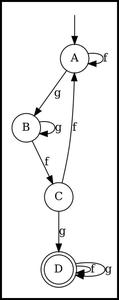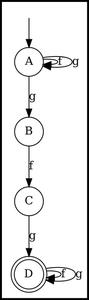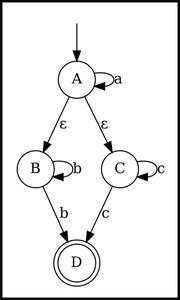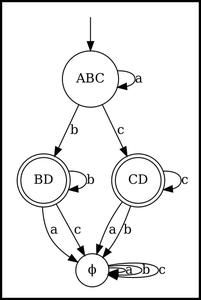# Converting Epsilon-NFA to DFA using Python and Graphviz

• Last Updated : 13 Feb, 2022

Finite Automata (FA) is a simple machine used to match patterns in an input string. Finite Automata is a quintuple i.e., it has five elements. In this article we are going to see how to convert epsilon-NFA to DFA using Python and Graphviz.

The quintuple of FA is represented as :The Five Elements are :

1. A finite set of States (Q)
2. A finite set of Input Alphabets ()
3. Start State ()
4. A finite set of Final States (F)
5. Transition Function ()

Finite Automata (FA) are of two types :

• Deterministic Finite Automata (DFA)
• Non-Deterministic Finite Automata (NFA)

### Deterministic Finite Automata (DFA)

Deterministic Finite Automata (DFA) is a FA where there is only one fixed state the machine can go to for an input alphabet as defined in the transition function.

DFA does not allow(null) alphabet which means that the machine will not change state if no alphabet is detected.

For a DFA,Example, DFA accepts all the strings made up of f’s and g’s which contains “gfg” as a substring.DFA

### Non-Deterministic Finite Automata (NFA)

Non-Deterministic Finite Automata (NFA) is a FA where the machine can go to more than one states for an input alphabet.

For an NFA,NFA which allowsmoves is called E-NFA or Epsilon-NFA.

NFA allowing(null) alphabet means that machine can change state even if no input alphabet is detected.

For an E-NFA,Example, NFA that accepts all the strings made up of f’s and g’s which contains “gfg” as a substring.NFA

Theis because, for every transition, there are 2 possibilities, to transit or not transit. And this is for every Q state. Sowill be the total possible configurations for each transition.

### Why the conversion?

Computers can understand FA in its basic form that is in DFA. But because of the features of NFA, we humans can understand NFA and better E-NFA with ease. So we need to convert the E-NFA to DFA.

### Steps For Converting E-NFA to DFA :– closure : It is the set of states to which we can go without any input i.e., withmoves.

Step 1: Find– closure of the start state of NFA and that will be the start state of DFA.

Step 2: Starting with this set, for each alphabet, evaluate– closure of the transition set for this alphabet.

Step 3: For each new set of closure set we come across, we will repeat Step 2 until no new set is left.

Step 4: The set in DFA which contains the final state of NFA will be the final set state.

#### For example,

Let the given E-NFA be :NFA

NFA :Q : {A, B, C, D}: {a, b, c}: A

F : {D}:

It is an NFA for language which accepts string of the type : {, where}

#### Steps to convert :

Step 1: Find– closure of the start state of NFA and that will be the start state of DFA.– closure of start state of NFA.– closure (A) : {A,B,C}

Steps 2,3: Starting with this set, for each alphabet, evaluate– closure of the transition set for this alphabet. and  For each new set of closure set we come across, we will repeat Step 2 until no new set is left.

Current set of states of DFA: {ABC}

• {ABC} -> a = A -> a : ABC
• {ABC} -> b = BD -> b : BD
• {ABC} -> c = CD -> c : CD

Current set of states of DFA: {ABC, BD, CD}

• {BD} -> a =• {BD} -> b = BD
• {BD} -> c =• {CD} -> a =• {CD} -> b =• {CD} -> c = CD

States of DFA, Q : {ABC, BD, CD,}

Transition Function:

Step 4: The set in DFA which contains the final state of NFA will be the final set state.

D was the final state in NFA. So all the states having D in its set will be final state in DFA.

So final states of DFA, F : {BD, CD}

DFA obtained :DFAis also known as dead state because there is no outgoing edge from it. So after machine arrives in the dead state, it cannot reach the final state.

Tools for implementation with code:

Graphviz: It is a python library for visualizing graph diagrams.

To install graphviz in python, run this command in the terminal:

pip install graphviz

Prerequisite:

Inputs

1. Number of States : no_state
2. Array of States : states
3. Number of Alphabets : no_alphabet
4. Array of Alphabets : alphabets
5. Start State : start
6. Number of Final States : no_final
7. Array of Final States : finals
8. Number of Transitions : no_transition
9. Array of Transitions : transitions
Transitions are of type : [From State, Alphabet, To State]

Utility :

1. Dictionary/Map to get Index from state : states_dict
2. Dictionary/Map to get Index from alphabet : alphabets_dict
3. Transition Table Dictionary to get array of ‘to’ states from ‘state’ and ‘alphabet’ pair : transition_table
4. Digraph object to store graph of the NFA : graph

Methods/Functions :

1. Constructor of the class NFA : __init__()
It initializes all the input variables and evaluates the utility variables values from input.
2. Get input from user : fromUser()
Class Method to get input from user.
3. Representation of the quintuple of NFA : __repr__()
4. Find Epsilon Closure of a state : getEpsilonClosure(state)
To find epsilon closure of a state, we maintain a stack to get what state to evaluate next and a dictionary to track which states have been evaluated.
We start a while loop from the start state, and find its epsilon transition states.
We push all this states to the stack (stack.push(stata)) and mark them in the dictionary (dict[state]=0).
We also mark this state complete in the dictionary (dict[state]=1).
For each next iteration, we pop the top of the stack each time and evaluate it if it is not evaluated by checking from the dictionary.
5. Find appropriate name of state for diagram of converted DFA from array of states : getStateName(state_list)
As we will get a list of states from the evaluation, to display in the DFA diagram, we need a proper name which will be concatenation of all the names in te set.
For ex : To state set is ={A,B,D} , then this function will return a string = “ABD”.
6. Check if the array contains a final state of NFA to find if the array will be final state in DFA : isFinalDFA(state_list)
This function checks if a list of states contains a state which is a final state in NFA, which in turn will tell if the set is final or not.
For ex : The set we are checking is = {A,B,D}, and D is a final state in NFA. So this function will return True for this input set. So this set will be final state in DFA.

Approach:

1. Make an object of NFA class: nfa, and initialize it with predefined values using a constructor or using user input.
2. Initialize the nfa.graph with nodes and edges according to the input values.
3. Display/Render the NFA graph.
4. Make another Digraph object to store values of the obtained DFA and to render the diagram: dfa.
5. Evaluate epsilon closure of all the states of NFA to not recalculate each time and store it in the dictionary with key-value pair as [state -> list of states of closure]: epsilon_closure{}
6. Make a stack to track which DFA state to evaluate next: dfa_stack[]
7. Add the epsilon closure of the start state of NFA as the start state of DFA.
8. Make a list to maintain all the states present in the DFA : dfa_states[]
9. Start a while loop which we continue till there are no new states in dfa_stack.
10. We pop the top of dfa_stack to evaluate the current set of states: cur_state.
11. We traverse through all the alphabets for the current set of states.
12. We make a set to maintain the epsilon closure of all the states in the current set : from_closure{}
13. If this set is not empty,
• We make another set to maintain the to_state set.
• If this set is not present in dfa_states, then we append it in dfa_stack and dfa_states.
• Then we add this node in dfa graph and add an edge between cur_state and to_state.
14. Else this set is empty, then
• This case is for dead state.
• If dead state not present in dfa, then we add the new state. And we make all transitions for all alphabets to itself so that the machine can never leave the dead state.
• We make transition of cur_state to this dead_state.
15. At last, all the states have been evaluated. So we will render/view this dfa graph.

Below is the full implementation:

## Python3

 # Conversion of epsilon-NFA to DFA and visualization using Graphviz from graphviz import Digraph class NFA:    def __init__(self, no_state, states, no_alphabet, alphabets, start,                 no_final, finals, no_transition, transitions):        self.no_state = no_state        self.states = states        self.no_alphabet = no_alphabet        self.alphabets = alphabets                 # Adding epsilon alphabet to the list        # and incrementing the alphabet count        self.alphabets.append('e')        self.no_alphabet += 1        self.start = start        self.no_final = no_final        self.finals = finals        self.no_transition = no_transition        self.transitions = transitions        self.graph = Digraph()         # Dictionaries to get index of states or alphabets        self.states_dict = dict()        for i in range(self.no_state):            self.states_dict[self.states[i]] = i        self.alphabets_dict = dict()        for i in range(self.no_alphabet):            self.alphabets_dict[self.alphabets[i]] = i                     # transition table is of the form        # [From State + Alphabet pair] -> [Set of To States]        self.transition_table = dict()        for i in range(self.no_state):            for j in range(self.no_alphabet):                self.transition_table[str(i)+str(j)] = []        for i in range(self.no_transition):            self.transition_table[str(self.states_dict[self.transitions[i]])                                  + str(self.alphabets_dict[                                      self.transitions[i]])].append(                                          self.states_dict[self.transitions[i]])     # Method to get input from User    @classmethod    def fromUser(cls):        no_state = int(input("Number of States : "))        states = list(input("States : ").split())        no_alphabet = int(input("Number of Alphabets : "))        alphabets = list(input("Alphabets : ").split())        start = input("Start State : ")        no_final = int(input("Number of Final States : "))        finals = list(input("Final States : ").split())        no_transition = int(input("Number of Transitions : "))        transitions = list()        print("Enter Transitions (from alphabet to) (e for epsilon): ")        for i in range(no_transition):            transitions.append(input("-> ").split())        return cls(no_state, states, no_alphabet, alphabets, start,                   no_final, finals, no_transition, transitions)     # Method to represent quintuple    def __repr__(self):        return "Q : " + str(self.states)+"\nΣ : "        + str(self.alphabets)+"\nq0 : "        + str(self.start)+"\nF : "+str(self.finals) + \            "\nδ : \n" + str(self.transition_table)     def getEpsilonClosure(self, state):               # Method to get Epsilon Closure of a state of NFA        # Make a dictionary to track if the state has been visited before        # And a array that will act as a stack to get the state to visit next        closure = dict()        closure[self.states_dict[state]] = 0        closure_stack = [self.states_dict[state]]         # While stack is not empty the loop will run        while (len(closure_stack) > 0):                       # Get the top of stack that will be evaluated now            cur = closure_stack.pop(0)                         # For the epsilon transition of that state,            # if not present in closure array then add to dict and push to stack            for x in self.transition_table[                    str(cur)+str(self.alphabets_dict['e'])]:                if x not in closure.keys():                    closure[x] = 0                    closure_stack.append(x)            closure[cur] = 1        return closure.keys()     def getStateName(self, state_list):               # Get name from set of states to display in the final DFA diagram        name = ''        for x in state_list:            name += self.states[x]        return name     def isFinalDFA(self, state_list):               # Method to check if the set of state is final state in DFA        # by checking if any of the set is a final state in NFA        for x in state_list:            for y in self.finals:                if (x == self.states_dict[y]):                    return True        return False  print("E-NFA to DFA") # INPUT# Number of States : no_state# Array of States : states# Number of Alphabets : no_alphabet# Array of Alphabets : alphabets# Start State : start# Number of Final States : no_final# Array of Final States : finals# Number of Transitions : no_transition# Array of Transitions : transitions nfa = NFA(    4,  # number of states    ['A', 'B', 'C', 'D'],  # array of states    3,  # number of alphabets    ['a', 'b', 'c'],  # array of alphabets    'A',  # start state    1,  # number of final states    ['D'],  # array of final states    7,  # number of transitions    [['A', 'a', 'A'], ['A', 'e', 'B'], ['B', 'b', 'B'],     ['A', 'e', 'C'], ['C', 'c', 'C'], ['B', 'b', 'D'],     ['C', 'c', 'D']]       # array of transitions with its element of type :    # [from state, alphabet, to state]) # nfa = NFA.fromUser() # To get input from user# print(repr(nfa)) # To print the quintuple in console # Making an object of Digraph to visualize NFA diagramnfa.graph = Digraph() # Adding states/nodes in NFA diagramfor x in nfa.states:    # If state is not a final state, then border shape is single circle    # Else it is double circle    if (x not in nfa.finals):        nfa.graph.attr('node', shape='circle')        nfa.graph.node(x)    else:        nfa.graph.attr('node', shape='doublecircle')        nfa.graph.node(x) # Adding start state arrow in NFA diagramnfa.graph.attr('node', shape='none')nfa.graph.node('')nfa.graph.edge('', nfa.start) # Adding edge between states in NFA from the transitions arrayfor x in nfa.transitions:    nfa.graph.edge(x, x, label=('ε', x)[x != 'e']) # Makes a pdf with name nfa.graph.pdf and views the pdfnfa.graph.render('nfa', view=True) # Making an object of Digraph to visualize DFA diagramdfa = Digraph() # Finding epsilon closure beforehand so to not recalculate each timeepsilon_closure = dict()for x in nfa.states:    epsilon_closure[x] = list(nfa.getEpsilonClosure(x))  # First state of DFA will be epsilon closure of start state of NFA# This list will act as stack to maintain till when to evaluate the statesdfa_stack = list()dfa_stack.append(epsilon_closure[nfa.start]) # Check if start state is the final state in DFAif (nfa.isFinalDFA(dfa_stack)):    dfa.attr('node', shape='doublecircle')else:    dfa.attr('node', shape='circle')dfa.node(nfa.getStateName(dfa_stack)) # Adding start state arrow to start state in DFAdfa.attr('node', shape='none')dfa.node('')dfa.edge('', nfa.getStateName(dfa_stack)) # List to store the states of DFAdfa_states = list()dfa_states.append(epsilon_closure[nfa.start]) # Loop will run till this stack is not emptywhile (len(dfa_stack) > 0):    # Getting top of the stack for current evaluation    cur_state = dfa_stack.pop(0)     # Traversing through all the alphabets for evaluating transitions in DFA    for al in range((nfa.no_alphabet) - 1):        # Set to see if the epsilon closure of the set is empty or not        from_closure = set()        for x in cur_state:            # Performing Union update and adding all the new states in set            from_closure.update(                set(nfa.transition_table[str(x)+str(al)]))         # Check if epsilon closure of the new set is not empty        if (len(from_closure) > 0):            # Set for the To state set in DFA            to_state = set()            for x in list(from_closure):                to_state.update(set(epsilon_closure[nfa.states[x]]))             # Check if the to state already exists in DFA and if not then add it            if list(to_state) not in dfa_states:                dfa_stack.append(list(to_state))                dfa_states.append(list(to_state))                 # Check if this set contains final state of NFA                # to get if this set will be final state in DFA                if (nfa.isFinalDFA(list(to_state))):                    dfa.attr('node', shape='doublecircle')                else:                    dfa.attr('node', shape='circle')                dfa.node(nfa.getStateName(list(to_state)))             # Adding edge between from state and to state            dfa.edge(nfa.getStateName(cur_state),                     nfa.getStateName(list(to_state)),                     label=nfa.alphabets[al])                     # Else case for empty epsilon closure        # This is a dead state(ϕ) in DFA        else:                       # Check if any dead state was present before this            # if not then make a new dead state ϕ            if (-1) not in dfa_states:                dfa.attr('node', shape='circle')                dfa.node('ϕ')                 # For new dead state, add all transitions to itself,                # so that machine cannot leave the dead state                for alpha in range(nfa.no_alphabet - 1):                    dfa.edge('ϕ', 'ϕ', nfa.alphabets[alpha])                 # Adding -1 to list to mark that dead state is present                dfa_states.append(-1)             # Adding transition to dead state            dfa.edge(nfa.getStateName(cur_state,),                     'ϕ', label = nfa.alphabets[al]) # Makes a pdf with name dfa.pdf and views the pdfdfa.render('dfa', view = True)

Output:DFA

The complexity of algorithm to convert NFA to DFA :

Time Complexity:Space Complexity:Where, Q = No. of States of NFA , N = No. of Alphabets of NFA.

My Personal Notes arrow_drop_up